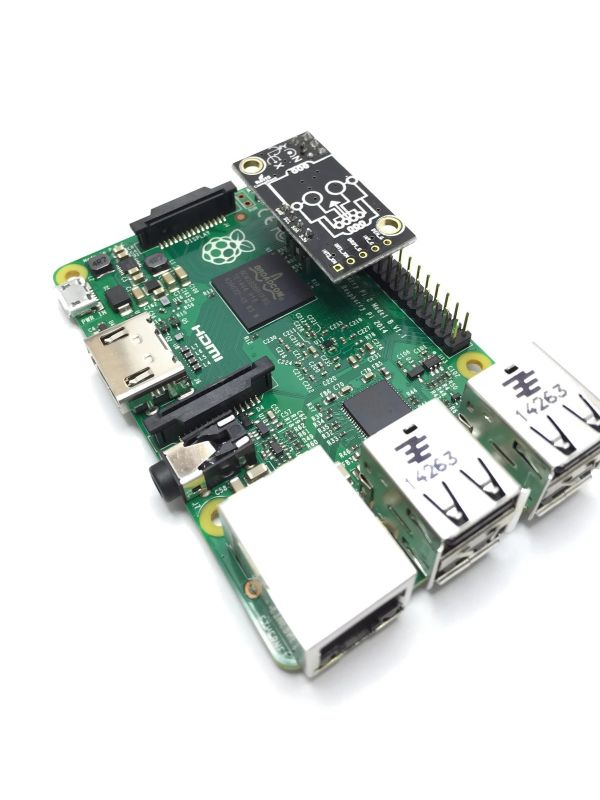# BerryIMU running on Raspberry Pi running Windows IoT

BerryIMU also works great with Windows IoT Core on the Raspberry Pi.

Our Git repository contains the source files needed to get the BerryIMU up and running on Windows IoT.

The code will print out the following values to the screen;

• Raw values from the gyroscope, accelerometer and magnetometer.
• Accelerometer calculated angles.
• Gyro tracked angles.
• Fused X and Y angles.

## Connecting BerryIMU to a Raspberry Pi

BrryIMU can connect via the jumper cables to the Raspberry Pi as shown below;Or BerryIMU can sit right on top of the GPIO pins on a Raspberry Pi. The first 6 GPIOs are used as shown below.# Get the Code

Download the BerryIMU code for Windows IoT from our GIT repository. The files you need are under the WindowsIoT-BerryIMU folder.

Once downloaded, double-click  the file WindowsIoT-BerryIMU.sln to open up the project in Visual Studio.

The project code outputs all of the needed values to the screen and a complementary filter is used to fuse the accelerometer and gyroscope angles.

We have a number of guides already documented on how to get the BerryIMU working with the Raspberry Pi.
https://ozzmaker.com/berryimu/
These are based on Raspbian, however the principals and math are the same for Windows Iot.

The final values which should be used are the fused X &Y angles and the tilt compensated heading.

The sensor on the BerryIMU is the LSM9DS0 and all the I2C registers for this sensor can be found in LSM9DS0.cs

The main code can be found in MainPage.xaml.cs#### Complementary Filter

A complementary filter is used to fuse the angles. Is summary, the complementary filter trusts the gyroscope for short periods and trusts the accelerometer for longer periods;

```CFangleX = AA * (CFangleX + (rate_gyr_x * DT / 1000)) + (1.0f - AA) * AccXangle;
CFangleY = AA * (CFangleY + (rate_gyr_y * DT / 1000)) + (1.0f - AA) * AccYangle;
```

Changing how much trust is given for each of the sensors can be changed by modify the complementary filter constant at the start of the code.

```const float AA = 0.03f;     // Complementary filter constant
```

#### Loop Speed

The loop speed is important as we need to know how much time has past to calculate the rotational degrees per second on the gyroscope.
A time delta is set at the start of the code.

```const int DT = 100;         //DT is the loop delta in milliseconds.
```

This is then used to specify a new timer method.

```periodicTimer = new Timer(this.TimerCallback, null, 0,DT);
```

Here you can see where DT is used to keep track of the gyroscope angle. You can also see it in the above calculation for the complementary filter.

```//Calculate the angles from the gyro
gyroXangle += rate_gyr_x * DT / 1000;
gyroYangle += rate_gyr_y * DT / 1000;
gyroZangle += rate_gyr_z * DT / 1000;
```

#### BerryIMU orientation

The calculations in the code are based on how the BerryIMU is orientated. If BerryIMU is upside down, then some of the angles need to be reversed. It is upside down when the skull logo is facing up(or to the sky).
If it is upside down, set the below value to true. Otherwise, set it to false.

```bool IMU_upside_down = true;
```

.

This site uses Akismet to reduce spam. Learn how your comment data is processed.